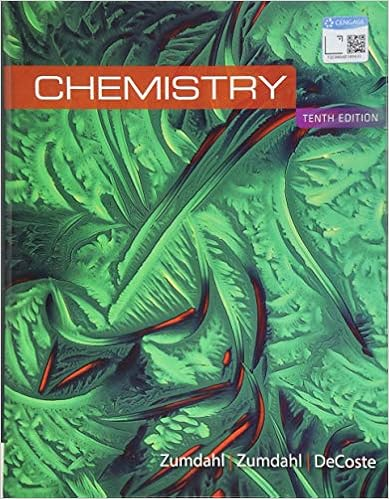E anode 271v 34v 237v reference chang 1811 1

• 4
• 75% (16) 12 out of 16 people found this document helpful

This preview shows page 1 - 3 out of 4 pages.

We have textbook solutions for you!
The document you are viewing contains questions related to this textbook.The document you are viewing contains questions related to this textbook.
Chapter 18 / Exercise 8
Chemistry
ZumdahlExpert Verified
- E˚ 2.71V = .34V - (-2.37V) anode (Reference: Chang 18.11)
We have textbook solutions for you!
The document you are viewing contains questions related to this textbook.The document you are viewing contains questions related to this textbook.
Chapter 18 / Exercise 8
Chemistry
ZumdahlExpert Verified
3. Predict whether Fe +3 can oxidize I to I 2 under standard-state conditions. (10 points) (Reference: Chang 18.13)
4. Consider the following half-reactions: MnO 4 ( aq ) + 8H + ( aq ) + 5 e Mn +2 ( aq ) + 4H 2 NO 3 ( aq ) + 4H + ( aq ) + 3 e NO( g ) + 2H 2 Predict whether NO 3 ions will oxidize Mn +2 to MnO 4 under standard state conditions. (8 points) O( l ) O( l ) anode
5. Which species in each pair is a better oxidizing agent under standard-state conditions? (16 points) 2
•••